# Sample GRE Multiple Choice Math Problem – Lost in Translation

On every GRE Math section, the test makers try to come up with a few extremely difficult problems that will leave even the cleverest students scratching their heads. The really evil part, though, is that even these problems can be solved in under a minute without a calculator – if you know what to do. This means that once you “figure out the trick,” these difficult problems become easy. So, while those test makers are busy cackling with sadistic glee, let’s see if we can’t beat them at their own game.

Consider the following problem:

The average of four numbers is five less than the average of the three numbers that remain after one has been eliminated. If the eliminated number is 2, what is the average of the four numbers?

A) 17

B) 22

C) 66

D) 68

E) 100

“What numbers?” you ask, “How am I supposed to find the averages of numbers that no one knows?!”

The GRE test makers love to make math questions harder by forcing you to work with variables instead of with actual numbers. Actual numbers are concrete, intuitive, and easy to understand, while variables are more abstract and difficult to grasp. At least that’s their theory. As you’ll see, variables aren’t actually harder than actual numbers. In fact, in some cases, problems that have many unknowns can actually be faster and easier to solve than problems with actual numbers, since there is less to figure out (because there’s less you can figure out). The main trick comes at the beginning, when we have to translate the words of a problem into a mathematical expression we can manipulate on our scratch paper.

First, the problem tells us that we have “the average of four numbers.” Well, how do you find the average of four numbers? You add them up and divide by 4. We can write this down if we pick variables to represent each of the four numbers. Let’s just go with a, b, c, and d.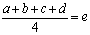Here, e represents the average of the four numbers (what we are trying to find). Next, the problem tells us that we take the average of 3 of the four numbers. This mean we have to leave out one of our variables. Since d is the last one, we’ll leave it out: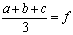The new average is represented by f. Now the problem also tells us that the variable that was left out was equal to 2, so we know that: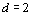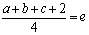The problem also tells us that the average of four numbers is five less than the average of the three numbers that remain after one has been eliminated. We have let e represent the average of the four numbers and f represent the average of the three numbers, so we could rephrase this as e is five less than f: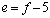So, now we still have all of these variables. We want to substitute variables for each other until we have one equation with one variable, because then we can solve for that variable and hopefully get closer to solving for e. Here’s one possible way to do that: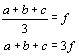We can now substitute 3f for a+b+c in the other equation: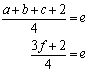We can also substitute f – 5 for e, since we know that e = f – 5: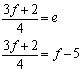Now we have one equation with only one variable, so we can solve for f: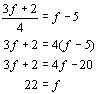Of course, we are trying to solve for e, so we should plug this into the equation e = f – 5: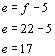Thus, the correct answer is choice A. Note, however, that if you had only solved for f, 22 was a choice as well. With this problem, it’s all about translating the words into variables and then moving the variables around until you can actually solve for one of them. This problem might be a little more time consuming than some of the others we’ve looked at in this series, but it can still be solved in under a minute without a calculator, if you know what to do. So you see, with patience and practice, even the hardest problems on the GRE become easy. As you do more practice problems you will get better and better at them – the test makers tend to use the same tricks over and over again. Check back here each week for more extra hard problems and the tricks you need to solve them! Also, remember that you can find out all the tricks from experts like me with a Test Masters course or private tutoring. Until  then, keep up the good work and happy studying!

This entry was posted in Example Problems and tagged , . Bookmark the permalink.